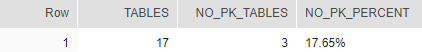## How many tables don't have primary keys (with percentage) in Snowflake

Article for:

This query listed tables without primary keys and this one shows how many of them there are and what is the percentage of total tables.

## Query

``````select sum.tables,
sum.tables - pk.pk_count as no_pk_tables,
round(100.0* (sum.tables - pk.pk_count) / sum.tables,2) ||'%'
as no_pk_percent
from (select count(*) as tables
from information_schema.tables tab
where tab.table_type = 'BASE TABLE')
as sum
left join(
select count(*) as pk_count
from information_schema.tables tab
join information_schema.table_constraints tco
on tab.table_schema = tco.table_schema
and tab.table_name = tco.table_name
and tco.constraint_type = 'PRIMARY KEY'
where tab.table_type = 'BASE TABLE'
) pk on true;
``````

## Columns

• tables - number of all tables in a database
• no_pk_tables - number of tables without a primary key
• no_pk_percent - percentage of tables without primary key in all tables

## Rows

Query returns just one row.

## Sample results